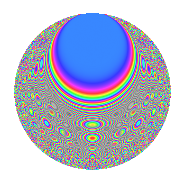# Properties

 Label 806.2.baLevel 806 Weight 2 Character orbit ba Rep. character $$\chi_{806}(119,\cdot)$$ Character field $$\Q(\zeta_{12})$$ Dimension 152 Newforms 1 Sturm bound 224 Trace bound 0

# Related objects

## Defining parameters

 Level: $$N$$ = $$806 = 2 \cdot 13 \cdot 31$$ Weight: $$k$$ = $$2$$ Character orbit: $$[\chi]$$ = 806.ba (of order $$12$$ and degree $$4$$) Character conductor: $$\operatorname{cond}(\chi)$$ = $$403$$ Character field: $$\Q(\zeta_{12})$$ Newforms: $$1$$ Sturm bound: $$224$$ Trace bound: $$0$$

## Dimensions

The following table gives the dimensions of various subspaces of $$M_{2}(806, [\chi])$$.

Total New Old
Modular forms 464 152 312
Cusp forms 432 152 280
Eisenstein series 32 0 32

## Trace form

 $$152q - 12q^{7} - 160q^{9} + O(q^{10})$$ $$152q - 12q^{7} - 160q^{9} + 12q^{11} - 4q^{12} + 4q^{14} + 76q^{16} - 12q^{17} + 16q^{18} - 28q^{19} + 20q^{21} - 12q^{26} + 12q^{28} - 12q^{29} + 20q^{31} + 28q^{33} - 24q^{34} - 4q^{35} - 28q^{37} - 72q^{38} + 20q^{39} + 36q^{41} - 36q^{42} - 16q^{43} - 12q^{44} - 84q^{45} - 24q^{46} - 8q^{47} + 36q^{49} + 24q^{51} - 8q^{52} - 12q^{53} + 8q^{57} - 12q^{58} - 24q^{59} - 12q^{61} - 36q^{62} + 4q^{63} + 120q^{65} + 32q^{66} - 52q^{67} + 48q^{69} + 48q^{70} - 76q^{71} - 16q^{72} - 52q^{73} + 48q^{74} + 92q^{75} + 4q^{76} + 96q^{77} + 12q^{78} + 12q^{79} + 120q^{81} + 28q^{84} - 24q^{85} - 36q^{86} + 64q^{87} - 12q^{89} - 44q^{91} + 120q^{93} - 8q^{94} - 124q^{97} + 40q^{98} + O(q^{100})$$

## Decomposition of $$S_{2}^{\mathrm{new}}(806, [\chi])$$ into irreducible Hecke orbits

Label Dim. $$A$$ Field CM Traces $q$-expansion
$$a_2$$ $$a_3$$ $$a_5$$ $$a_7$$
806.2.ba.a $$152$$ $$6.436$$ None $$0$$ $$0$$ $$0$$ $$-12$$

## Decomposition of $$S_{2}^{\mathrm{old}}(806, [\chi])$$ into lower level spaces

$$S_{2}^{\mathrm{old}}(806, [\chi]) \cong$$ $$S_{2}^{\mathrm{new}}(403, [\chi])$$$$^{\oplus 2}$$